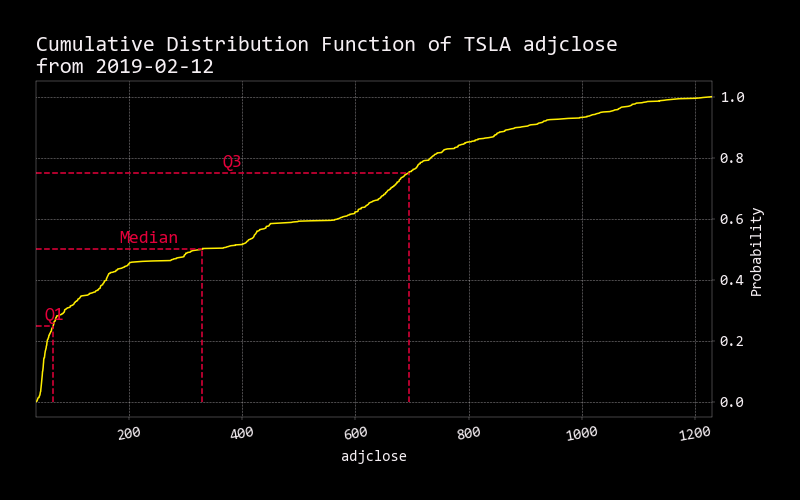# Cdf

``````usage: cdf [--export {csv,json,xlsx}] [-h]
``````

Explanation excerpt from: https://www.probabilitycourse.com/chapter3/3_2_1_cdf.php

The cumulative distribution function (CDF) of a random variable is another method to describe the distribution of random variables. The advantage of the CDF is that it can be defined for any kind of random variable (discrete, continuous, and mixed).

The cumulative distribution function (CDF) of random variable X is defined as:

Contextual Example: I toss a coin twice. Let X be the number of observed heads. Find the CDF of X

``````optional arguments:
--export {csv,json,xlsx}
Export dataframe data to csv,json,xlsx file (default: )
-h, --help            show this help message (default: False)
``````